# Pharmacy

The pharmacy adds to 3 liters of 95-percent alcohol 5 liters of 38.04 percent alcohol. How much percent alcohol the pharmacy got?

p =  59.4063 %

### Step-by-step explanation:

3•0.95+5•0.3805 = p/100 •(3+5)

8p = 475.25

p = 1901/32 = 59.40625

Our simple equation calculator calculates it.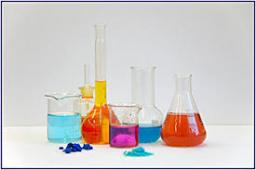Did you find an error or inaccuracy? Feel free to write us. Thank you!Tips to related online calculators
Looking for a statistical calculator?
Do you have a linear equation or system of equations and looking for its solution? Or do you have a quadratic equation?
Tip: Our volume units converter will help you with the conversion of volume units.

## Related math problems and questions:

• Alcohol mixtureThree liters of 96 percent alcohol set up a certain amount of distilled water to form 54-percent alcohol. How many liters of distilled water was used?
• Alcohol mixing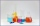How much 64% of the alcohol must be poured into 6 liters of 85% alcohol to produce 79% alcohol?
• Pouring alcohol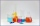100 liters of alcohol has 70% How many liters of water needs to be added to have 60% alcohol?
• Vinegar 2How many percentages of vinegar solution will we get if we mix 3.5 liters of 5.8% and 5 liters of 7.6% vinegar?
• Mixture 2How many liters of water must be added to 7 liters of a 20% solution to obtain a 10% solution?
• Alcohol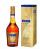How many cubic centimeters of 96% alcohol is needed to obtain 0.75 liters of 40% alcohol?
• Alcohol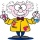6l 48% alcohol, how much 52% alcohol must be added to give 50% alcohol?
• AlcoholHow many 55% alcohol we need to pour into 14 liters 75% alcohol to get p3% alcohol? How many 65% alcohol we get?
• Alcohol 2Two types of alcohol one 63% and second 75% give 20 liters of 69% alcohol. How many liters of each type are in the mixture?
• Disinfecting solutionHow much distilled water is necessary to pour into 500 ml of 33% hydrogen peroxide solution to obtain 3% disinfecting solution?
• CuSO4 mixtureHow many grams of solid CuSO4 we have to add to 450g of 15% CuSO4 solution to produce a 25% solution?
• Vinegar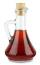What percentage vinegar we get if we mix 1 if dm³ eight percent vinegar with 1.5 dm³ six percent vinegar?
• Alcohol solutionsWe have to produce 2 liters of 60% alcohol from 55% and 80%. How many of which ones will we use in the solution?
• Solutions, mixturesHow many liters of 70% solution must we add to 5 liters of 30% solution to give us a 60% solution?
• Average score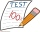In a math exam, two students scored 95, six students scored 90, 3 students scored 80, and one students scored 50. What was the average score for the class?
• SolutionsHow much 60% solution and how much 35% solution is needed to create 100 l of 40% solution?
• PeroxideHow many ml should we pour 30% of peroxide (H2O2) into 100ml H2O to give a 20% solution?